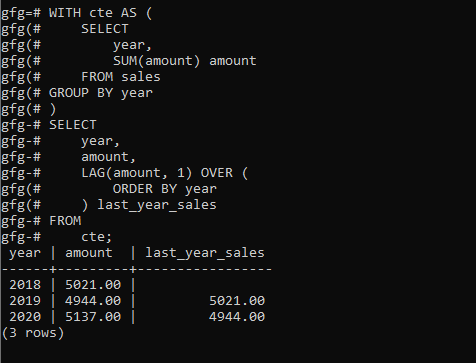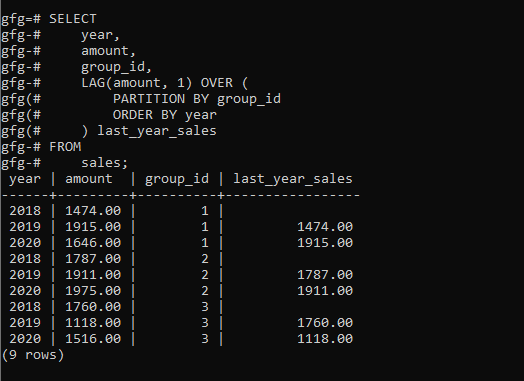GeeksforGeeks App
Open AppBrowser
Continue

# PostgreSQL – LAG Function

In PostgreSQL, the LAG() function is used to access a row that comes exactly before the current row at a specific physical offset. The LAG() comes in handy while comparing the values of the current row with the previous row.

The following shows the syntax of LAG() function:

```Syntax:
LAG(expression [, offset [, default_value]])
OVER (
[PARTITION BY partition_expression, ... ]
ORDER BY sort_expression [ASC | DESC], ...
)```

Let’s analyze the above syntax:

• The expression is used to set a comparison basis for the comparison of current and exactly previous rows at a specified offset. It can any among the following, be a column, an expression, or a subquery.
• The offset is a positive integer that is used to set in the query the number of rows that come before the current row. The offset can be an expression, a subquery, or a column. If it is not specified, it defaults to 1.
• The LAG() function will return the default_value when the offset goes beyond the scope of the partition.
• The LAG() function is applied on the partitions created by the PARTITION BY clause.If partition is not specified, the function treats the whole result set as a single partition.
• The ORDER BY clause sets the order of the rows in each partition to which the LAG() function is applied.

Example 1:

Let’s set up a new table for the demonstration named sales:

```CREATE TABLE sales(
year SMALLINT CHECK(year > 0),
group_id INT NOT NULL,
amount DECIMAL(10, 2) NOT NULL,
PRIMARY KEY(year, group_id)
);```

```INSERT INTO
sales(year, group_id, amount)
VALUES
(2018, 1, 1474),
(2018, 2, 1787),
(2018, 3, 1760),
(2019, 1, 1915),
(2019, 2, 1911),
(2019, 3, 1118),
(2020, 1, 1646),
(2020, 2, 1975),
(2020, 3, 1516);```

Here the LAG() function to return the sales amount of the current year and the previous year:

```WITH cte AS (
SELECT
year,
SUM(amount) amount
FROM sales
GROUP BY year
)
SELECT
year,
amount,
LAG(amount, 1) OVER (
ORDER BY year
) last_year_sales
FROM
cte;```

Output:Example 2:

This example uses the LAG() function to compare the sales of the current year with the sales of the previous year of each product group:

```SELECT
year,
amount,
group_id,
LAG(amount, 1) OVER (
PARTITION BY group_id
ORDER BY year
) last_year_sales
FROM
sales;```

Output:My Personal Notes arrow_drop_up